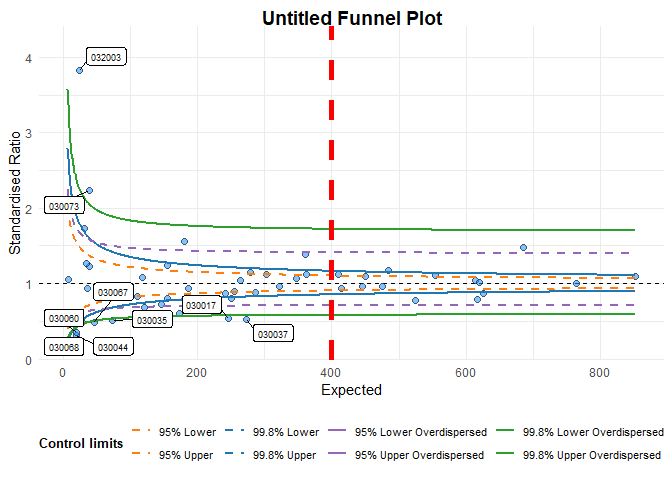# Changing funnel plot options

## Setup

library(FunnelPlotR)

This brief vignette shows how to go about changing some of the parameters available in the funnelplotr package. Firstly, lets set up some data, the same as the README and other vignette:

library(COUNT)
data(medpar)
medpar$provnum<-factor(medpar$provnum)
medpar$los<-as.numeric(medpar$los)

# Logistic model to predict LOS, LOS is quite overdispersed
mod<- glm(los ~ hmo + died + age80 + factor(type), family="poisson", data=medpar)

#Get predicted value for ratio
medpar$prds<- predict(mod, newdata = medpar, type="response") # Draw plot, returning just the plot object funnel_plot(denominator=medpar$prds, numerator=medpar$los , group = medpar$provnum, limit=99 ,label = "outlier"
, draw_unadjusted = TRUE)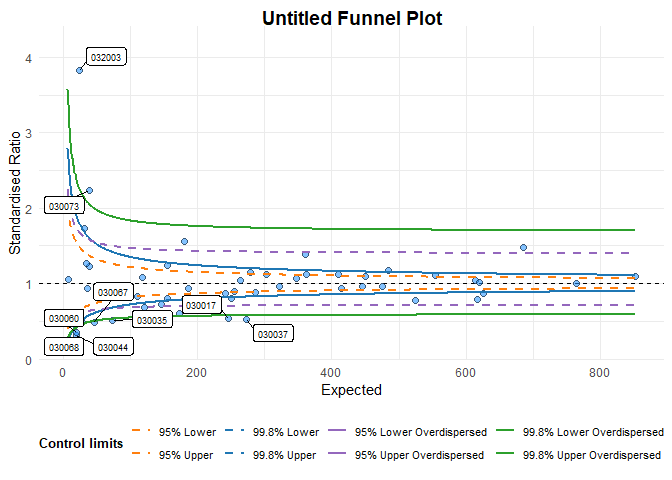#> A funnel plot object with 54 points of which 9 are outliers.
#> Plot is adjusted for overdispersion.

## Highlighting a data point

You can pick out data point(s) using the highlight option. Here we we use the example above to highlight the hospital labels ‘030002’ in the data set.

# Draw plot, returning just the plot object
funnel_plot(denominator=medpar$prds,numerator=medpar$los
, group = medpar$provnum, limit=99 ,label = "outlier" , draw_unadjusted = TRUE, highlight="030002")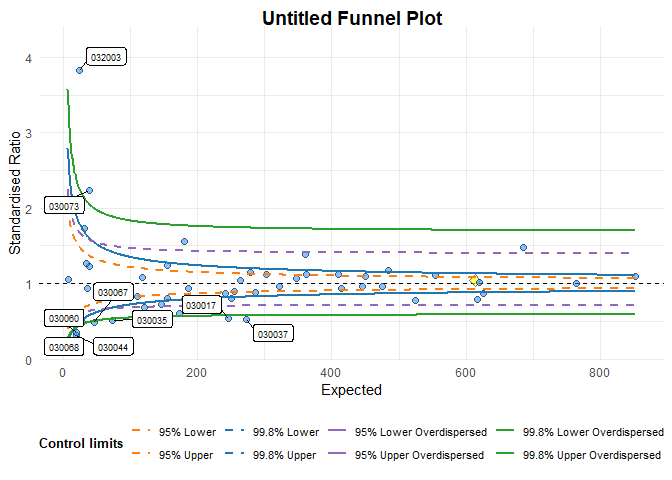#> A funnel plot object with 54 points of which 9 are outliers. #> Plot is adjusted for overdispersion. ## Themes You can alter themes in the funnelplotr packages by using the theme argument. There are a couple of options included with the package funnel_clean (the default) and funnel_grey, but you can write your own theme using any valid ggplot2 theme and pass it to the plot. funnel_plot(denominator=medpar$prds,numerator=medpar$los , group = medpar$provnum, limit=99 ,label = "outlier"
, draw_unadjusted = TRUE, theme = funnel_grey() )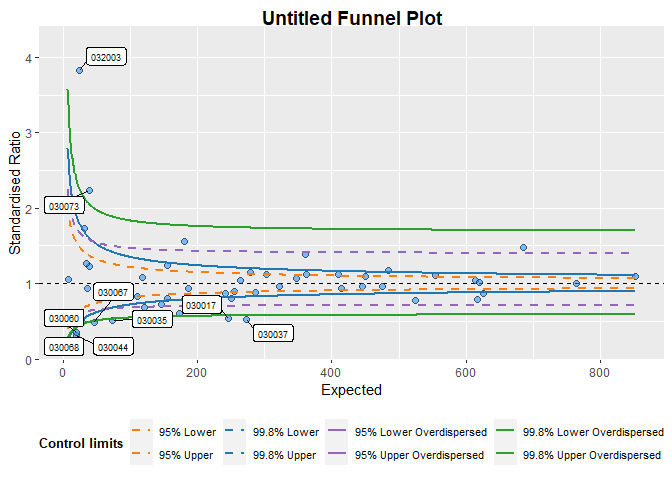#> A funnel plot object with 54 points of which 9 are outliers.
#> Plot is adjusted for overdispersion.

If you are not familiar with themes, you can create a theme with the theme function from ggplot2. I will create a new theme, including the funnel_grey theme, but with some different (exaggerated) elements.

library(ggplot2)

new_funnel_theme <-
funnel_grey()+
theme(plot.title = element_text(face="bold", colour="red", size=6), # Change plot title
legend.background = element_rect(fill="brown"), # Alter legend background colour
axis.title.y = element_text(angle=0)  #Rotate y axis label
)

funnel_plot(denominator=medpar$prds,numerator=medpar$los
, group = medpar$provnum, limit=99 ,label = "outlier" , draw_unadjusted = TRUE, theme = new_funnel_theme)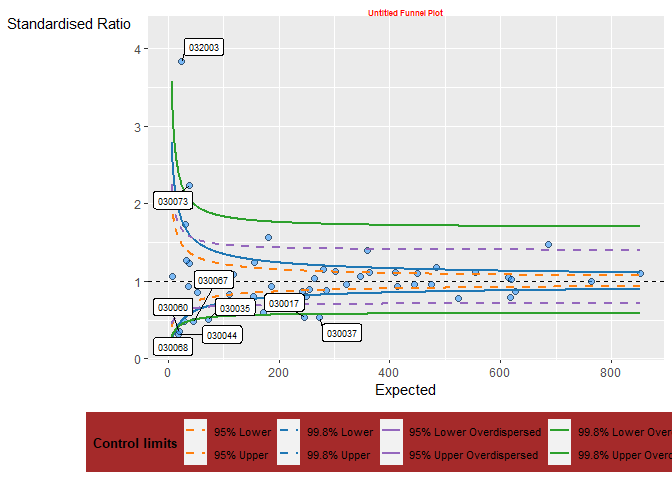#> A funnel plot object with 54 points of which 9 are outliers. #> Plot is adjusted for overdispersion. ## Plot colours You can change the colours of the limits. I am aiming to allow better colour theme support in future versions, but at present they are supplied as a vector of eight hex colours to the plot_cols argument, in the order 95% Poisson (lower/upper), 99.8% Poisson (lower/upper), 95% OD (lower/upper) and 99.8% OD (lower/upper). At present all eight values are required, whether you are plotting all eight limits or not. I have deliberately avoided using red and green colours as defaults because it encourages value judgements about ‘good’ v.s. ‘bad’ which may be unreasonable for a given data set. Default colours are paired for both low and high: c("#FF7F0EFF", "#FF7F0EFF", "#1F77B4FF","#1F77B4FF", "#9467BDFF", "#9467BDFF", "#2CA02CFF", "#2CA02CFF") Here I will change the upper 95% Poisson limit to black (“#000000”): funnel_plot(denominator=medpar$prds,numerator=medpar$los , group = medpar$provnum, limit=99 ,label = "outlier"
, draw_unadjusted = TRUE, theme = funnel_grey(),
plot_cols = c("#FF7F0EFF", "#000000", "#1F77B4FF","#1F77B4FF", "#9467BDFF", "#9467BDFF", "#2CA02CFF", "#2CA02CFF"))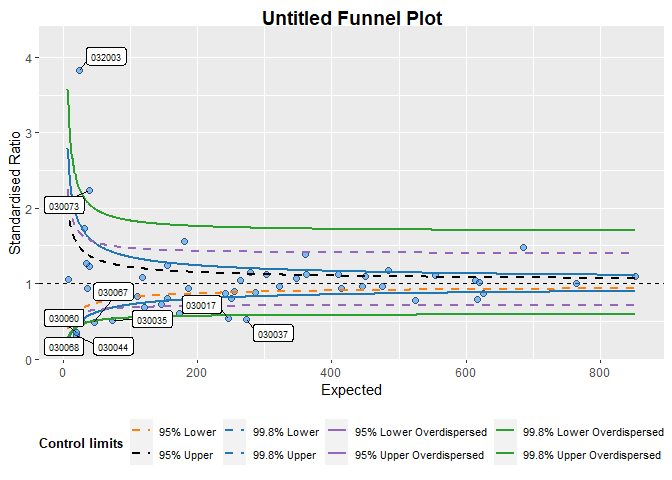#> A funnel plot object with 54 points of which 9 are outliers.
#> Plot is adjusted for overdispersion.

## Changing scales

funnelplotr automatically sets it’s scales from the data you present to it but, on occasions, the scale rules might fall down for a particular dataset, or you may want to fix the plot to a particular scale. You can do this using the xrange and yrange arguments. Each takes a vector of two values, the minimum and the maximum:

## Changing labels
funnel_plot(denominator=medpar$prds,numerator=medpar$los
, group = medpar$provnum, limit=99 ,label = "outlier" , draw_unadjusted = TRUE, xrange=c(0, 400), yrange=c(0,2)) #> Warning: The xrange argument deprecated; please use the x_range argument #> instead. For more options, see the help: ?funnel_plot #> Warning: The yrange argument deprecated; please use the y_range argument #> instead. For more options, see the help: ?funnel_plot #> Warning: Removed 17 rows containing missing values (geom_point).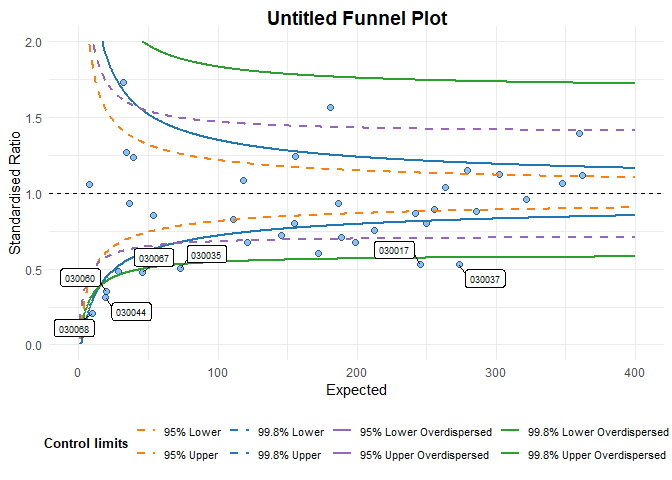#> A funnel plot object with 54 points of which 9 are outliers. #> Plot is adjusted for overdispersion. ggplot2 will warn you, as above, if you are excluding any points from your plot. ## Changing labels You can change the plot labels and axis labels easily using the options: title, x_label and y_label. funnel_plot(denominator=medpar$prds,numerator=medpar$los , group = medpar$provnum, limit=99 ,label = "outlier"
, draw_unadjusted = TRUE, title = "Vignette funnel plot"
, x_label = "x-axis", y_label = "y-axis")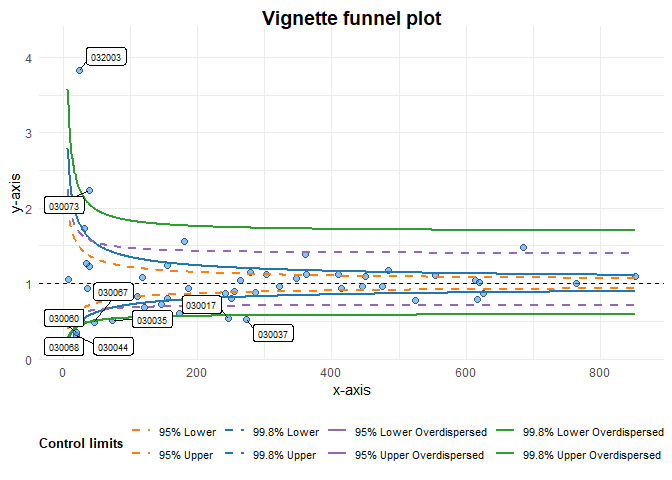#> A funnel plot object with 54 points of which 9 are outliers.
#> Plot is adjusted for overdispersion.

There are different labelling options for the data points too, using the label option. The default is to label outliers, but you can turn labels off, label the highlighted points, or both the highlighted points and the outliers (‘both’)

funnel_plot(denominator=medpar$prds,numerator=medpar$los
, group = medpar$provnum, limit=99 , draw_unadjusted = TRUE, title = "Vignette funnel plot" , x_label = "x-axis", y_label = "y-axis" , highlight= "030002", label = "highlight")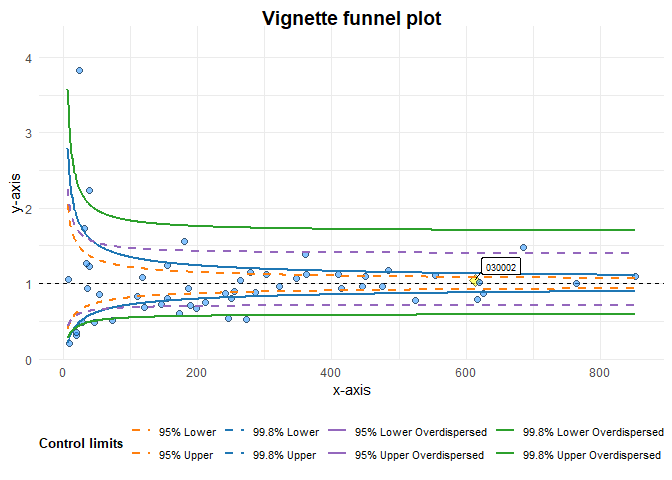#> A funnel plot object with 54 points of which 9 are outliers. #> Plot is adjusted for overdispersion. ## Cutting out the ggplot object Since funnelplotr uses ggplot2, you could always extract the plot and alter it manually like any other ggplot2 object. The easiest way is to extract it with plot(). Below we’ll add a (completely useless) vertical line to demonstrate adding more elements: # Original funnel plot object fp <- funnel_plot(denominator=medpar$prds,numerator=medpar$los , group = medpar$provnum, limit=99, label = "outlier"
geom_vline(aes(xintercept=400), linetype = "dashed", colour="red", size=2)